Courses

# Doc: Nernst Equation and Electrochemical Cell and Gibbs Energy of Reaction Class 12 Notes | EduRev

## Chemistry Class 12

Created by: Mohit Rajpoot

## Class 12 : Doc: Nernst Equation and Electrochemical Cell and Gibbs Energy of Reaction Class 12 Notes | EduRev

The document Doc: Nernst Equation and Electrochemical Cell and Gibbs Energy of Reaction Class 12 Notes | EduRev is a part of the Class 12 Course Chemistry Class 12.
All you need of Class 12 at this link: Class 12

Nernst Equation

Walter nernst derived a relation between cell potential and concentration or Reaction quotient.

ΔG = ΔGº RT ln Q ..........(i)

where, ΔG and ΔGº are free energy and standard free energy change, 'Q' is reaction quotient.

- ΔG = nFE and -ΔGº = nFEº

Thus from Eq. (i), we get - nFE = - nFEº + RT lnQ

At 25ºC, above equation may be written as E = Eº -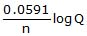Where 'n' represents number of moles of electrons involved in process.

E, Eº are e.m.f. and standard e.m.f. of the cell respectively.

In general, for a redox cell reaction involving the transference of n electrons

aA + bB → cC + dD, the EMF can be calculated as:

ECell = EºCell  -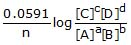Thermodynamic Treatment of Nernest Equation

(i) Prediction and feasibility of spontaneity of a cell reaction.

Let us see whether the cell (Daniell) is feasible or not; i.e. whether Zinc will displace copper or not.

Zn | (s) | ZnSO4(sol) || CuSO4(sol) | Cu(s)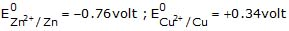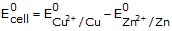= 0.34 - (- 0.76) = +1.10 volt

Since E0 = +ve, hence the cell will be feasible and zinc will displace copper from its salt solution. In the other words zinc will reduce copper.

(ii) Determination of equilibrium constant :  We know, that

E = E0 -..........(1)

At equilibrium, the cell potential is zero because cell reactions are balanced, i.e. E = 0

From Eq. (i), we have

0 = E0 -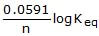or Keq = anti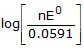(iii) Heat of Reaction inside the cell : Let n Faraday charge flows out of a cell of e.m.f. E, then

- ΔG = nFE ..........(i)

Gibbs Helmholtz equation (from thermodynamics) may be given as,

ΔG = ΔH + T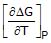..........(ii)

From Eqs. (i) and (ii), we have

- nFE = ΔH +T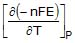= DH - nFT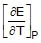ΔH = - nFE + nFT(iv) Entropy change inside the cell : We know that
G = H - TS or ΔG = ΔH - TΔS ..........(i)

where ΔG = Free energy change ; ΔH = Enthalpy change and ΔS = entropy change.

According to Gibbs Helmholtz equation,

ΔG = ΔH +T............(ii)

From Eqs. (i) and (ii), we have

- TΔS = Tor ΔS = -or ΔS = nFwhereis called temperature coefficient of cell e.m.f.

Different types of half-cells and their reduction potential

(1) Gas - Ion Half Cell :

In such a half cell, an inert collector of electrons, platinum or grphite is in contact with gas and a solution containing a specified ion. One of the most important gas-ion half cell is the hydrogen-gas-hydrogen ion half cell. In this cell, purified H2 gas at a constant pressure is passed over a platinum electrode which is in contact with an acid solution.

H (aq) e-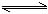1/2 H2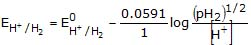(2) Metal-Metal Ion Half Cell :

This type of cell consist of a metal M is contact with a solution containing M ions.

M (aq) + neM(s)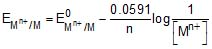(3) Metal-Insoluble Salt-Anion Half Cell :

In this half cell, a metal coated with its insoluble salt is in contact with a solution containing the anion of the insoluble salt. eg.-Silver-Silver Chloride Half Cell :

This half cell is represented as Cl-/AgCl/Ag. The equilibrium reaction that occurs at the electrode is

AgCl(s) + e-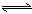Ag+(s) + Cl-(aq)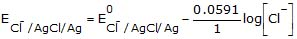,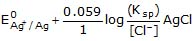(4) Oxidation-reduction Half Cell :

This type of half cell is made by using an inert metal collector, usually platinum, immersed in a solution which contains two ions of the same element in different states of oxidation. eg. Fe - Fe half cell.

Fe3+ (aq)  + e-Fe2+ (aq)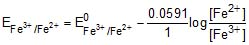Concentration cell

The cells in which electrical current is produced due to transport of a substance from higher to lower concentration. Concentration gradient may arise either in electrode material or in electrolyte. Thus there are two types of concentration cell.

(i) Electrode Gas concentration cell :

Pt, H2(P1)|H+ (C)|H 2(P2), Pt

Here, hydrogen gas is bubbled at two different partial pressures at electrode dipped in the solution of same electrolyte.

Cell Process : 1/2H2(p1) → H (c) e- (Anode process)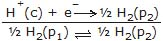E =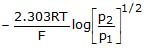or E = -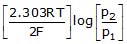,

At 25ºC, E = -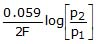For spontanity of such cell reaction p1 > p2

(2) Electrolyte concentration cells:

Zn(s) |ZnSO4(C1)||ZnSO4(C 2) | Zn(s)

In such cells, concentration gradient arise in electrolyte solutions. Cell process may be given as,

Zn(s) → Zn(C1)  + 2e- (Anodic process)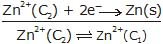(Over all process)

From Nernst equation, we have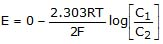or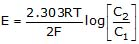For spontanity of such cell reaction, C2 > C1 .

Commercial voltaic cells

Batteries can be classified as primary and secondary. Primary batteries can not be returned to their original state by recharging, so when the reactants are consumed, the battery is "dead" and must be discarded. Secondary batteries are often called storage batteries or rechargeable batteries. The reactions in these batteries can be reversed; thus, the batteries can be recharged.

Primary batteries:

Dry Cells and Alkaline Batteries

Zinc serves as the anode, and the cathode is a graphite rod placed down the center of the device. These cells are often called "dry cells" because there is no visible liquid phase. However, water is present, so the cell contains a moist paste of NH4Cl, ZnCl2 and MnO2. The moisture is necessary because the ions present must be in a medium in which they can migrate from one electrode to the other. The cell generates a potential of 1.5 V using the following half-reactions.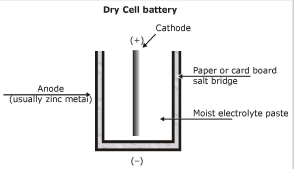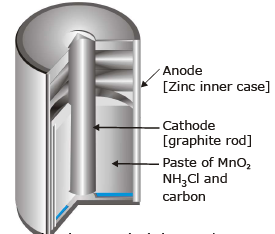Cathode, reductions :
2NH4 (aq)  + 2e- → 2NH3(g)  + H2(g)
Anode, oxidation :
Zn(s) → Zn2+ (aq) + 2e-

The two gases formed at the cathode will build up pressure and could cause the cell to rupture. This problem is avoided, however, by two other reactions that take place in the cell. Ammonia molecules bind to Zn ions, and hydrogen gas is oxidized by MnO2 to water.

Zn2+ (aq) + 2NH3(g) 2Cl-(aq) → Zn(NH3) + Cl2(s)

2MnO2(s)  + H2(g) → Mn2O3(s)  + H2O(l)

Leclanche cells were widely used because of their low cost, but they have several disadvantages. If current is drawn from the battery rapidly, the gaseous products cannot be consumed rapidly enough, so the cell resistance rises, and the voltage drops. In addition, the zinc electrode and ammonium ions are in contact in the cell, and these chemicals react slowly.

Recall that zinc reacts with acid to form hydrogen. The ammonium ion, NH4 (aq), is a weak Bronsted acid and reacts slowly with zinc. Because of this reaction, these voltaic cells cannot be stored indefinitely. When the zinc outer shell deteriorates, the battery can leak acid and perhaps damage the appliance in which it is contained.

At the present time alkaline batteries are used the chemistry of alkaline cells is quite similar to that in a Leclanche cell, except that the material inside the cell is basic (alkaline). Alkaline cells use the oxidation of zinc and the reduction of MnO2 to generate a current, but NaOH or KOH is used in the cell instead of the acidic salt NH4Cl.

Cathode, reductions:
2MnO2(s) + H2O(l) + 2e- → Mn2O3(s) + 2OH-(aq)

Anode, Oxidation:
Zn(s) + 2OH-(aq) → ZnO(s) + H2O(l) + 2e-

Alkaline cells, which produce 1.54 V (approximately the same voltage as the Leclanche cell), have the further advantage that the cell potential does not decline under high current loads because no gases are formed.

Secondary or Rechargeable batteries

An automobile battery-the lead storage battery - is probably the best-known rechargeable battery figure. The 12-V version of this battery contains six voltaic cells, each generating about 2V. The lead storage battery can produce a large initial current, an essential feature when starting an automobile engine.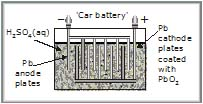When the cell supplies electrical energy, the lead anode is oxidized to lead (II) sulfate, an insoluble substance that adheres to the electrode surface. The two electrons produced per lead atom move through the external circuit to the cathode, where PbO2 is reduced to Pb ions that in presence of H2SO4, also form lead (II) sulfate.

Cathode, reduction:
PbO2(s) + 4H (aq) + SO42- (aq) + 2e- → PbSO4(s) + 2H2O(l)

Anode, oxidation:
Pb(s) + SO42- (aq) → PbSO4(s) + 2e-

------------------------------------------------------------------------------------

Net ionic equation
Pb(s) + PbO2(s) + 2H2SO4(aq) → 2PbSO4(s) + 2H2O(l)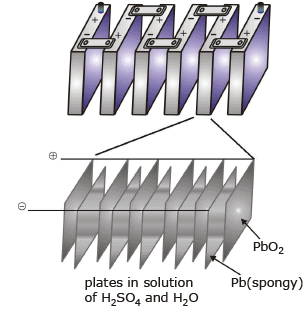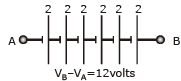Nickel-Cadimium ("Ni-Cad") batteries, used in variety of cordless appliances such as telephones, video camcorders, and cordless power tools, are lightweight and rechargeable. The chemistry of the cell utilizes the oxidation of cadmium and the reduction of nickel (III) oxide under basic conditions. As with the lead storage battery, the reactants and products formed when producing a current are solids that adhere to the electrodes.

Cathde, reduction :
NiO(OH)(s) + H2O(l) + e- → Ni(OH)2(s) + OH-(aq)
Anode, Oxidation:
Cd(s) + 2OH- → Cd(OH)2(s) + 2e-

Electrochemical cell and Gibbs Energy of the Reaction

Electrical work done in one second is equal to electrical potential multiplied by total charge passed. If we want to obtain maximum work from a galvanic cell then charge has to be passed reversibly. The reversible work done by a galvanic cell is equal to decrease in its Gibbs energy and therefore if the emf of the cell is E and nF is the amount of charge passed and ΔrG is the Gibbs energy of the reaction, then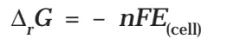It may be remembered that E(cell) is an intensive parameter but ΔrG is an extensive thermodynamic property and the value depends on n. Thus, if we write the reaction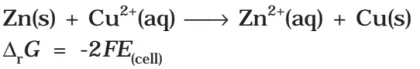But when we write the reaction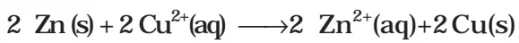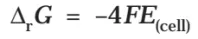If the concentration of all the reacting species is unity, then E(cell)
Thus, from the measurement of ECell we can obtain an important thermodynamic quantity, ΔrG, standard Gibbs energy of the reaction.From the latter we can calculate equilibrium constant by the equation: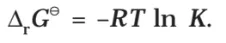Offer running on EduRev: Apply code STAYHOME200 to get INR 200 off on our premium plan EduRev Infinity!

,

,

,

,

,

,

,

,

,

,

,

,

,

,

,

,

,

,

,

,

,

;